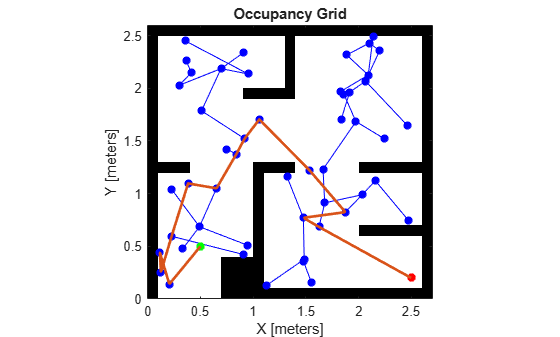# plannerPRM

Since R2022a

## Description

The probabilistic roadmap path planner constructs a roadmap without start and goal states. Use the `plan` function to find an obstacle-free path between the specified start and goal states. If the `plan` function does not find a connected path between the start and the goal states, it returns an empty path.

## Creation

### Syntax

``planner = plannerPRM(stateSpace,stateVal)``
``planner = plannerPRM(___,Name=Value)``

### Description

example

````planner = plannerPRM(stateSpace,stateVal)` creates a PRM planner from a state space object, `stateSpace`, and a state validator object, `stateVal`. The state space of `stateVal` must be the same as `stateSpace`. `stateSpace` and `stateVal` also sets the `StateSpace` and `StateValidator` properties, respectively, of the planner.```
````planner = plannerPRM(___,Name=Value)` sets properties using one or more name-value pair arguments in addition to the input arguments in the previous syntax. You can specify the `MaxNumNodes` or `MaxConnectionDistance` properties as name-value pairs.```

## Properties

expand all

State space for the planner, specified as a state space object. You can use state space objects such as `stateSpaceSE2`, `stateSpaceDubins`, and `stateSpaceReedsShepp`. You can also customize a state space object using the `nav.StateSpace` object.

State validator for the planner, specified as a state validator object. You can use state validator objects such as `validatorOccupancyMap` and `validatorVehicleCostmap`. You can also customize a state validator object using the `nav.StateValidator` object.

Maximum number of nodes in the graph, specified as a positive scalar. By increasing this value, the chance of finding a path increases while also increasing the computation time for the path planner.

Maximum distance between two connected nodes, specified as a positive scalar in meters. Nodes with distance greater than this value will not be connected in the graph.

## Object Functions

 `copy` Create deep copy of plannerPRM object `graphData` Retrieve graph as digraph object `plan` Plan path between start and goal states on roadmap

## Examples

collapse all

Create an occupancy map from an example map and set the map resolution as 10 cells/meter.

```map = load("exampleMaps.mat").simpleMap; map = occupancyMap(map,10);```

Create a state space and update the state space bounds to be the same as the map limits.

```ss = stateSpaceSE2; ss.StateBounds = [map.XWorldLimits; map.YWorldLimits; [-pi pi]];```

Create a state validator with `stateSpaceSE2` using the map and set the validation distance.

```sv = validatorOccupancyMap(ss,Map=map); sv.ValidationDistance = 0.01;```

Create a `plannerPRM` object.

`planner = plannerPRM(ss,sv);`

Retrieve graph as a digraph object.

`graph = graphData(planner);`

Extract nodes and edges from graph.

```edges = table2array(graph.Edges); nodes = table2array(graph.Nodes);```

Specify the start and goal states.

```start = [0.5 0.5 0]; goal = [2.5 0.2 0];```

Plot map and graph.

```show(sv.Map) hold on plot(nodes(:,1),nodes(:,2),"*","Color","b","LineWidth",2) for i = 1:size(edges,1) % Samples states at distance 0.02 meters. states = interpolate(ss,nodes(edges(i,1),:), ... nodes(edges(i,2),:),0:0.02:1); plot(states(:,1),states(:,2),"Color","b") end plot(start(1),start(2),"*","Color","g","LineWidth",3) plot(goal(1),goal(2),"*","Color","r","LineWidth",3)```

Plan a path with default settings. Set the `rng` seed for repeatability.

```rng(100,"twister"); [pthObj, solnInfo] = plan(planner,start,goal);```

Visualize the results.

```if solnInfo.IsPathFound interpolate(pthObj,1000); plot(pthObj.States(:,1),pthObj.States(:,2), ... "Color",[0.85 0.325 0.098],"LineWidth",2) else disp("Path not found") end hold off```Load a 3-D occupancy map of a city block into the workspace. Specify the threshold to consider cells as obstacle-free.

```mapData = load("dMapCityBlock.mat"); omap = mapData.omap; omap.FreeThreshold = 0.5;```

Inflate the occupancy map to add a buffer zone for safe operation around the obstacles.

`inflate(omap,1)`

Create an SE(3) state space object with bounds for state variables.

`ss = stateSpaceSE3([0 220;0 220;0 100;inf inf;inf inf;inf inf;inf inf]);`

Create a 3-D occupancy map state validator using the created state space. Assign the occupancy map to the state validator object. Specify the sampling distance interval.

```sv = validatorOccupancyMap3D(ss, ... Map = omap, ... ValidationDistance = 0.1);```

Create a probabilistic roadmap path planner with increased maximum connection distance.

`planner = plannerPRM(ss,sv);`

Specify start and goal poses.

```start = [40 180 25 0.7 0.2 0 0.1]; goal = [150 33 35 0.3 0 0.1 0.6];```

Configure the random number generator for repeatable result.

`rng(1,"twister");`

Plan the path.

`[pthObj,solnInfo] = plan(planner,start,goal);`

Visualize the planned path.

```show(omap) axis equal view([-10 55]) hold on % Start state scatter3(start(1,1),start(1,2),start(1,3),"g","filled") % Goal state scatter3(goal(1,1),goal(1,2),goal(1,3),"r","filled") % Path plot3(pthObj.States(:,1),pthObj.States(:,2),pthObj.States(:,3), ... "r-",LineWidth=2)```L.E. Kavraki, P. Svestka, J.C. Latombe, M.H. Overmars, "Probabilistic roadmaps for path planning in high-dimensional configuration spaces," IEEE Transactions on Robotics and Automation, Vol. 12, No. 4, pp. 566-580, Aug 1996.